Checkout JEE MAINS 2022 Question Paper Analysis : Checkout JEE MAINS 2022 Question Paper Analysis :

# I.E. Irodov Solutions on Constant Electric Field in Vacuum

Constant Electric Field in Vacuum is an important chapter in Physics that mainly deals with electric field and properties. The chapter comprises various numerical based on the syllabus and also the practical applications. I.E. Irodov Physics provides the solution to different varieties of numerical. Constant Electric Field in Vacuum is a necessary resource element that is needed by all students to score good marks in JEE Main and other entrance examinations. It is one of the best ways to study and practice for physics examinations to score high. The problems asked here are mainly based on electric field, current, speed, voltage and so on.

Each solution is explained stepwise and with formulas, equations, graphs, and diagrams wherever required. The concept explained here is crystal clear and goes hand to hand with the syllabus. These problems connect the theoretical concepts with practical and also enhance their application in daily life and activities. Students aiming for a high score in competitive examination must solve all the given problems for easy understanding and to have a grip over the syllabus and concept.

One can get access to all sorts of problems based on each and every formula, equations and method. The method adopted to solve practical problems is easily understandable by the students. It is a complete capsule for the students who are curious to solve more and more numerical and practical problems related to the subject. Each chapter contains various sections for making the concepts simpler and easier to understand. Every problem is explicitly explained with a detailed solution.

### I.E. Irodov Solutions on Constant Electric Field in Vacuum

1. Calculate the ratio of the electrostatic to gravitational interaction forces between two electrons, between two protons. At what value of the specific charge q/m of a particle would these forces become equal (in their absolute values) in the case of interaction of identical particles?

Solution:

1.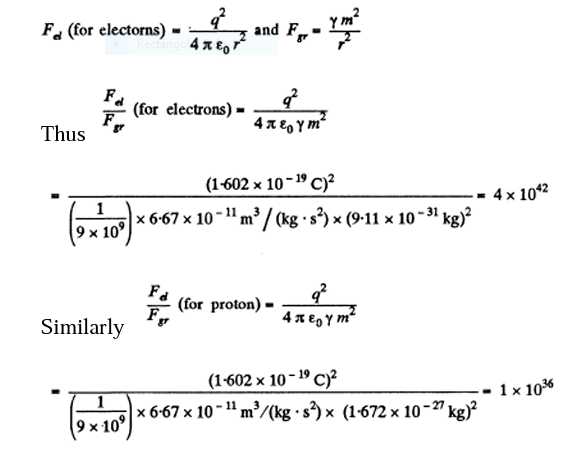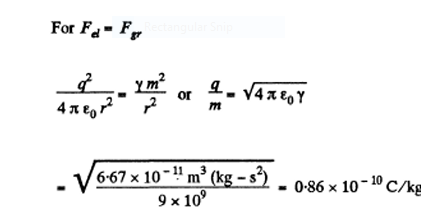= 0.86 x 10-10 C/kg

2. What would be the interaction force between two copper spheres, each of mass 1m, separated by the distance 1 m, if the total electronic charge in them differed from the total charge of the nuclei by one per cent?

Solution:

1. Total number of atoms in the sphere of mass 1 gm =

$$\frac{1}{63.54} \times 6.023 \times 10^{23}$$

So the total nuclear charge =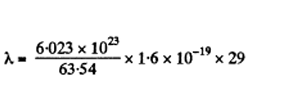Now the charge on the sphere = Total nuclear charge - Total electronic charge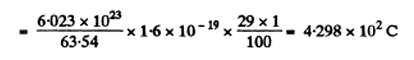Hence force of interaction between these two spheres,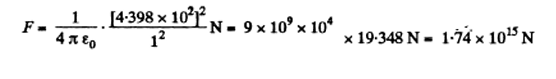= 1.74 X 1015

3. Two small equally charged spheres, each of mass m, are suspended from the same point by silk threads of length 1. The distance between the spheres x << 1. Find the rate dolt with which the charge leaks off each sphere if their approach velocity varies as

$$v=\frac{a}{\sqrt{x}}$$
where a is a constant.

Solution:

1. Let the balls be deviated by an angle θ, from the vertical when separation between them equals x.

Applying Newton’s second law of motion for any one of the sphere,

we get,

T cos θ = mg--- (1)

And T sin θ = Fe …………….(2)

From the Eqs. (1) and (2)

tan θ = Fe/mg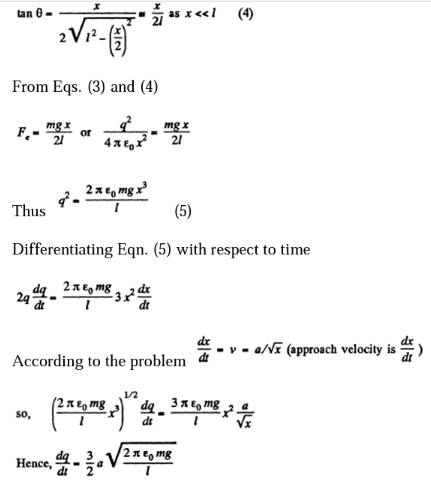4. Two positive charges q1 and q2 are located at the points with radius vectors r1 and r2. Find a negative charge q3 and a radius vector r3 of the point at which it has to be placed for the force acting on each of the three charges to be equal to zero.

Solution:

1. Let us choose coordinate axes as shown in the figure and fix three charges, q1 and q2 and q3 having position vectors r1, r2 and r3 respectively.

Now, for the equilibrium of q3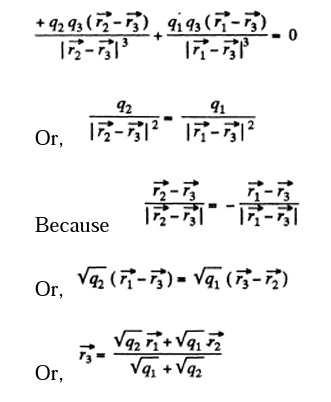Also for the equilibrium of q1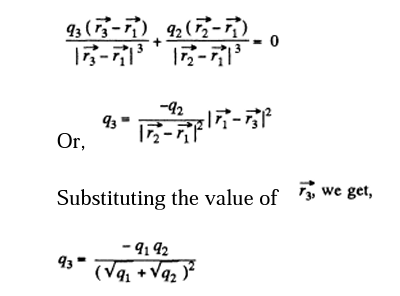5. A thin wire ring of radius r has an electric charge q. What will be the increment of the force stretching the wire if a point charge q0 is placed at the ring's centre?

Solution:

1. When the charge

$$q_{o}$$
is placed at the centre of the ring, the wire gets stretched and the extra tension, produced in the wire, will balance the electric force due to the charge q0. Let the tension produced in the wire, after placing the charge
$$q_{o}$$
be T. From Newton’s second law in projection form
$$F_{n}=mw_{n}$$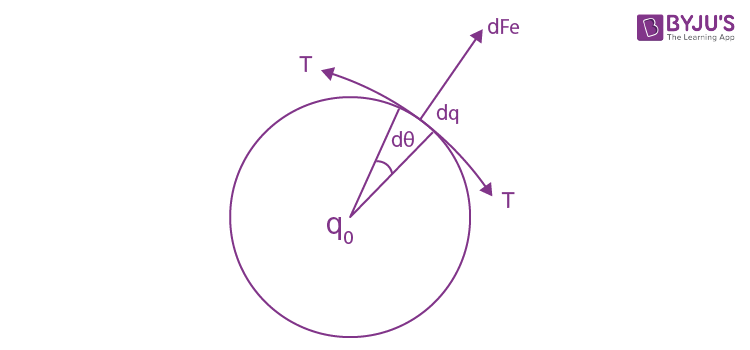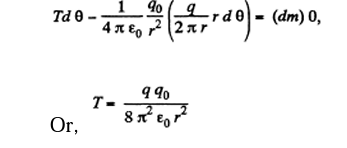6. A positive point charge 50 μC is located in the plane xy at the point with radius vector

$$r_{o}$$
= 2i + 3j, where i and j are the unit vectors of the x and y axes. Find the vector of the electric field strength E and its magnitude at the point with radius vector r = 8i — 5j. Here r0 and r are expressed in metres.

Solution:

1. Sought field strength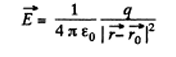= 4.5 kV/m on putting the values.

7. Point charges q and —q are located at the vertices of a square with diagonals 2l as shown in Figure Find the magnitude of the electric field strength at a point located symmetrically with respect to the vertices of the square at a distance x from its centre.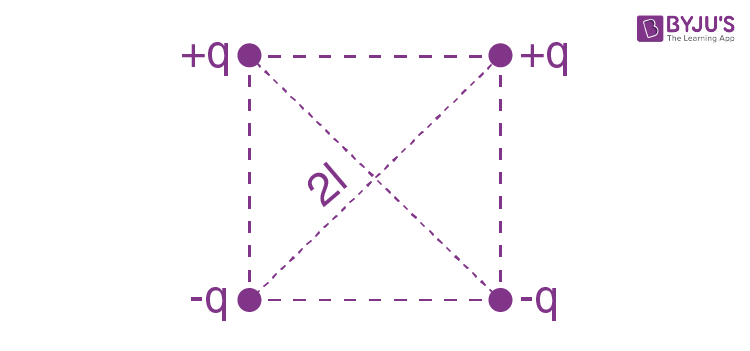Solution:

1. Let us fix the coordinate system by taking the point of intersection of the diagonals as the origin and let

$$\vec{k}$$
be directed normally, emerging from the plane of the figure.

Hence the sought field strength: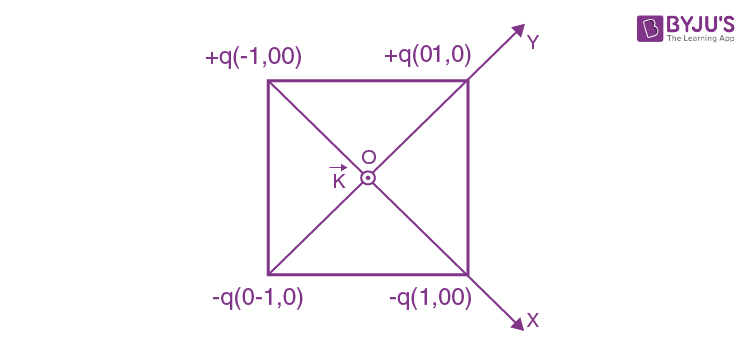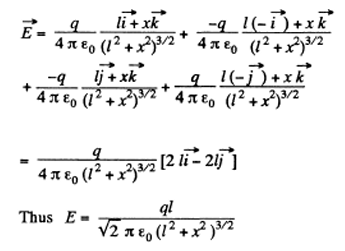8. A thin half q = 0.70 ring of radius R = 20 cm is uniformly charged with a total charge nC. Find the magnitude of the electric field strength at the curvature centre of this half ring.

Solution:

1. From the symmetry of the problem, the sought field.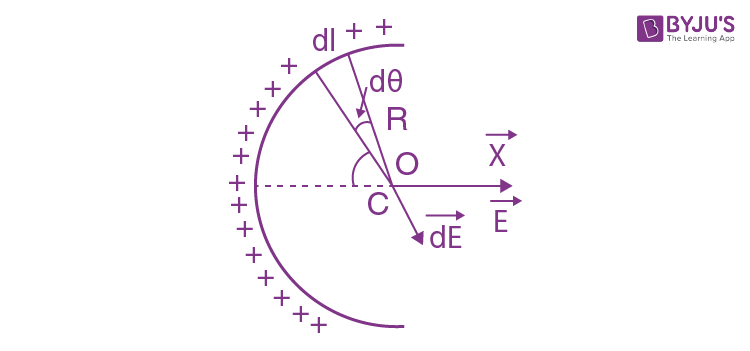$$E = \int d E_{x}$$

Where the projection of field strength along x-axis due to an elemental charge is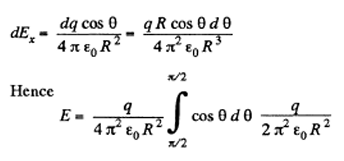9. A thin wire ring of radius r carries a charge q. Find the magnitude of the electric field strength on the axis of the ring as a function of distance l from its centre. Investigate the obtained function at 1≫ r. Find the maximum strength magnitude and the corresponding distance l. Draw the approximate plot of the function E(l).

Solution:

1. From the symmetry of the condition, it is clear that, the field along the normal will be zero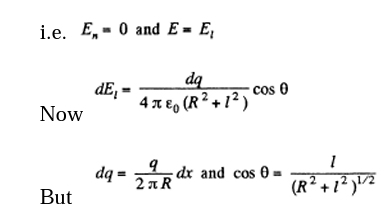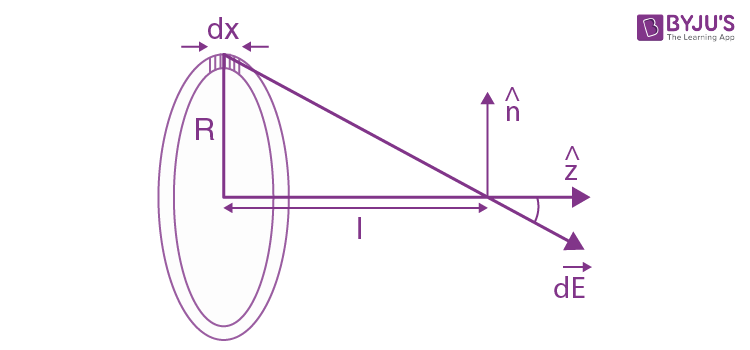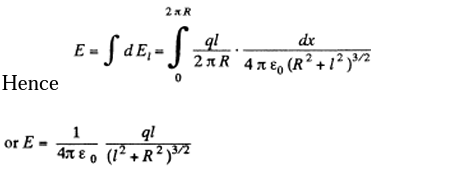And for l ≫ R, the ring behaves like a point charge, reducing the field to the value,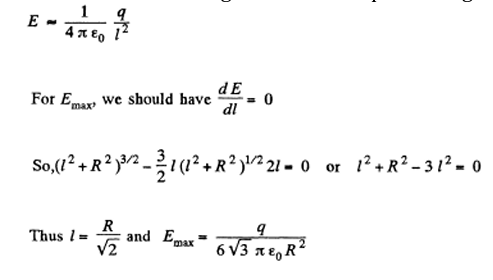10. A point charge q is located at the centre of a thin ring of radius R with uniformly distributed charge — q. Find the magnitude of the electric field strength vector at the point lying on the axis of the ring at a distance x from its centre, if x ≫ R.

Solution:

1. The electric potential at a distance a: from the given ring is given by,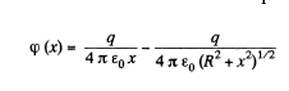Hence, the field strength along x-axis (which is the net field strength in 'our case),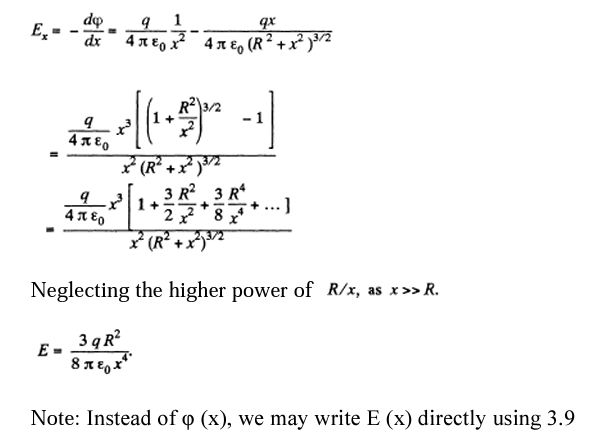11. A system consists of a thin charged wire ring of radius R and a very long uniformly charged thread oriented along the axis of the ring, with one of its ends coinciding with the centre of the ring. The total charge of the ring is equal to q. The charge of the thread (per unit length) is equal to λ. Find the interaction force between the ring and the thread.

Solution:

1. From the solution of 3.9, the electric field strength due to ring at a point on its axis (say x-axis) at distance x from the centre of the ring is given by:

$$E(x)=\frac{qx}{4\pi \varepsilon _{o}(R^{2}+ x^{2})^{\frac{3}{2}}}$$

And from symmetry, every point on the axis is directed along the x-axis. Let us consider an element (dx) on the thread that carries the charge (λdx). The electric force experienced by the element in the field of the ring.

$$dF=(\lambda dx)(Ex)=\frac{\lambda qx dx}{4\pi \varepsilon _{o}(R^{2}+ x^{2})^{\frac{3}{2}}}$$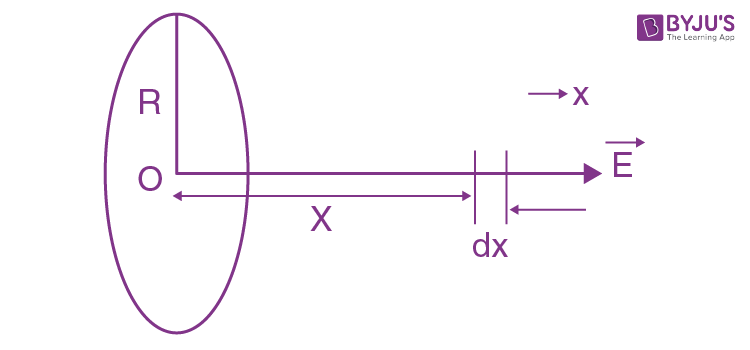Thus the sought interaction

$$F\int_{0}^{\infty }=\frac{\lambda qx dx}{4\pi \varepsilon _{o}(R^{2}+ x^{2})^{\frac{3}{2}}}$$

On integrating we get,

$$F=\frac{\lambda q}{4\pi \varepsilon _{o}R}$$

12. A thin non conducting ring of radius R has a linear charge density

$$\lambda =\lambda _{0}$$
cos φ, where
$$\lambda _{0}$$
is a constant, φ is the azimuthal angle. Find the magnitude of the electric field strength

1. (a) at the centre of the ring;

2. (b) on the axis of the ring as a function of the distance x from its centre. Investigate the obtained function at x ≫ R.

Solution:

1. (a) The given charge distribution is shown in Fig. The symmetry of this distribution implies that vector

$$\vec{E}$$
at the point O is directed to the right, and its magnitude is equal to the sum of the projection onto the direction of
$$\vec{E}$$
of vectors
$$\vec{dE}$$
from elementary charges dq. The projection of vector
$$\vec{dE}$$
onto vector
$$\vec{E}$$
is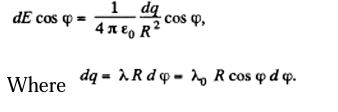Integrating (1) over φ between 0 and 2 π we find the magnitude of the vector E:

$$E=\frac{\lambda_{0}}{4\pi \varepsilon _{o}R}\int_{0}^{2x}\cos ^{2}\phi d\phi =\frac{\lambda_{0}}{4\pi \varepsilon _{o}R}$$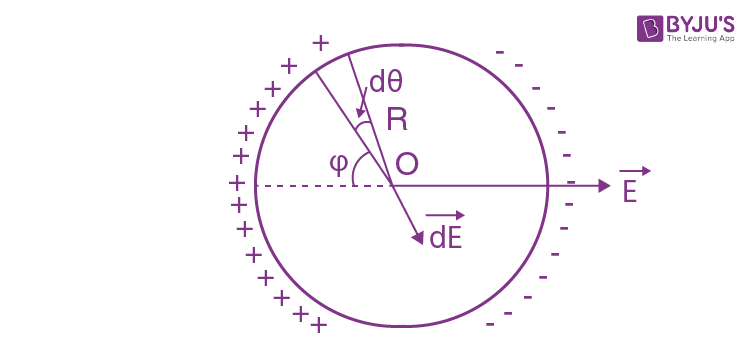It should be noted that this integral is evaluated in the most simple way if we take into account that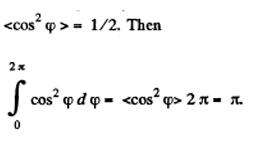(b) Take an element S at an azimuthal angle φ from the x an angle dφ at the centre.The elementary field at P due to the element is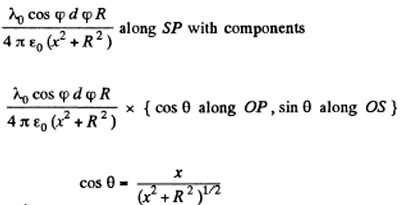Where

The component along OP vanishes on integration as

$$\int_{0}^{2x}\cos \phi d\phi =0$$

The component along axis, the element subtending OS can be broken into the parts along OX and OY with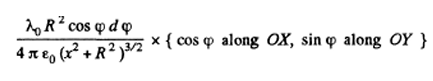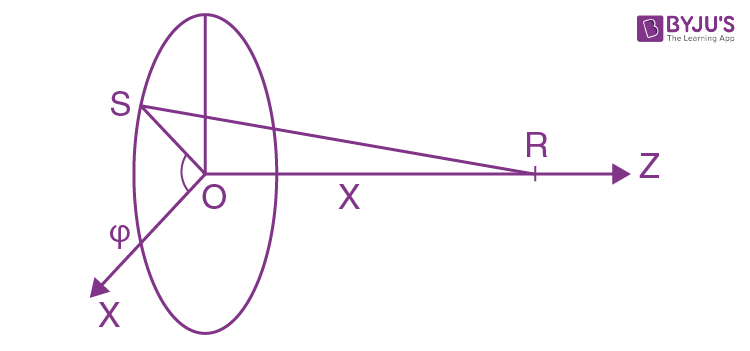On integration,the part along OY vanishes. Finally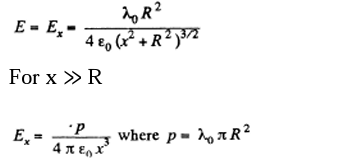13. A thin straight rod of length 2a carrying a uniformly distributed charge q is located in a vacuum. Find the magnitude of the electric field strength as a function of the distance r from the rod's centre along the straight line

1. (a) perpendicular to the rod and passing through its centre;

2. (b) coinciding with the rod's direction (at the points lying outside the rod). Investigate the obtained expressions at r ≫ a.

Solution:

1. (a) It is clear from symmetry considerations that vector

$$\vec{E}$$

be directed as shown in the figure. This shows the way of solving this problem: we must find the component dEr of the field created by the element dl of the rod, having the charge dq and then integrate the result over all the elements of the rod. In this case

$$dE_{r}=dE \cos \alpha =\frac{1}{4\pi \varepsilon _{0}}\frac{\lambda dl}{r^{2}_{o}}\cos \alpha$$

Where

$$\lambda =\frac{q}{2a}$$
is the linear charge density. Let us reduce this equation of the form convenient for integration. Figure shows that

$$dl \cos \alpha = r_{o}d\alpha$$
and
$$r_{0}=\frac{r}{cos \alpha }$$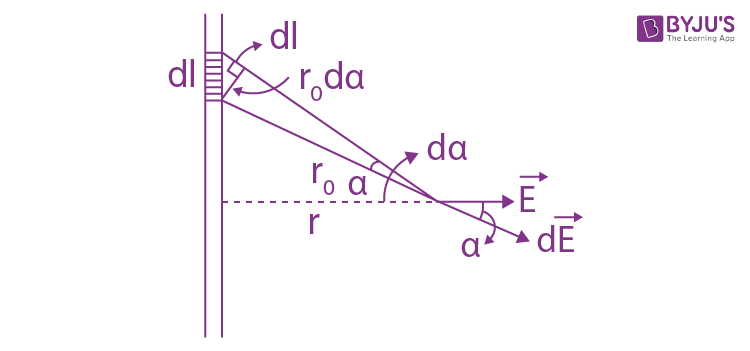Consequently

$$dE_{r}=\frac{1}{4\pi \varepsilon _{0}}\frac{\lambda r_{o}d\alpha }{r^{2}_{0}}= \frac{\lambda }{4\pi \varepsilon _{0}r}\cos \alpha d\alpha$$

We can integrate the above equation as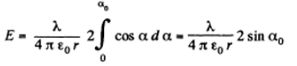$$\sin \alpha _{0}=\frac{q}{\sqrt{a^{2}+ r^{2}}}$$

Hence,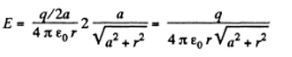Note that in case,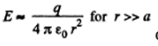of all field of a point charge.

(b) Let, us consider the element of length dl at a distance l from the centre of the rod, as shown in the figure. If the element lies on the side, shown in the diagram, and on other side. if it lies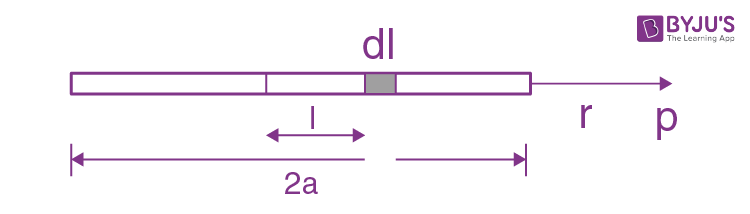Then field at P, due to this element.

$$dE=\frac{\lambda dl}{4\pi \varepsilon _{0}(r-l)^{2}}$$

If the element lies on the side, shown in the diagram, and

$$dE=\frac{\lambda dl}{4\pi \varepsilon _{0}(r+l)^{2}}$$
if it lies on the other side.

Thus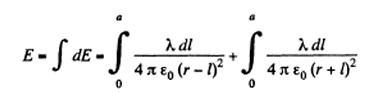On integration and substituting

$$\lambda =\frac{q}{2a}$$
we get

$$E= \frac{q}{4\pi \varepsilon _{0}}\frac{1}{(r^{2}-a^{2})}$$

For r>>>a

$$E\approx \frac{q}{4\pi \varepsilon _{0}}\frac{1}{r^{2}}$$

14. A very long straight uniformly charged thread carries a charge λ. per unit length. Find the magnitude and direction of the electric field strength at a point which is at a distance y from the thread and lies on the perpendicular passing through one of the thread's ends.

Solution:

1. The problem is reduced to finding Ex and Ey viz . the projections of

$$\vec{E}$$
where it is assumed that λ > 0. Let us start with Ex. The contribution to Ex. from the charge element of the segment dx is

$$dE_{x}= \frac{1}{4\pi \varepsilon _{0}}\frac{\lambda dx}{r^{2}}\sin \alpha$$
------(i)

Let us reduce this expression to the form convenient for integration. In our case, dx = r Ja/cos α, r = y/cosα.Then

$$dE_{x}= \frac{1}{4\pi \varepsilon _{0}y}\sin \alpha d\alpha$$

Integrating the above expression over

$$\alpha$$
between o and
$$\frac{\pi }{2}$$

$$E_{x}= \frac{\lambda }{4\pi \varepsilon _{0}y}$$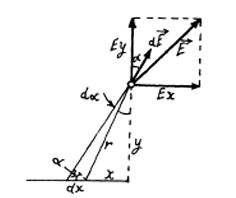In order to find the projection Ey it is sufficient to recall that it differs from dEdx in that

$$\sin \alpha$$
in (1) is simply replaced by
$$\cos \alpha$$
. This gives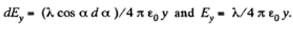Now we have obtained teresting result

$$E_{x}$$
=
$$E_{y}$$
independently if y

That is

$$\vec{E}$$
is oriented at an angle of 45 degrees to the rod.

The modulus of

$$\vec{E}$$
is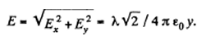15. A thread carrying a uniform charge λ per unit length has the configurations shown in Figure a and b. Assuming a curvature radius R to be considerably less than the length of the thread, find the magnitude of the electric field strength at the point O.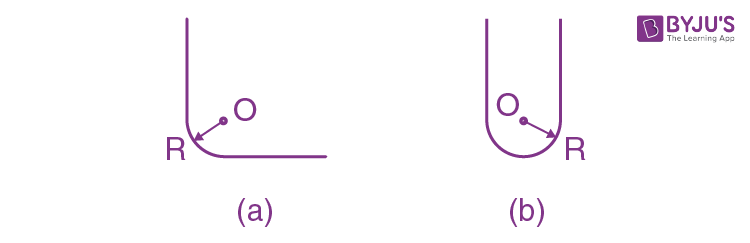Solution:

1. (a) Using the solution of Q.14, the net electric field strength at the point O due to straight parts of the thread equals zero. For the curved part (arc) let us derive a general expression i.e. Let us calculate the field strength at the centre of arc of radius R and linear charge density λ and which subtends angle

$$\Theta _{o}$$
at the centre.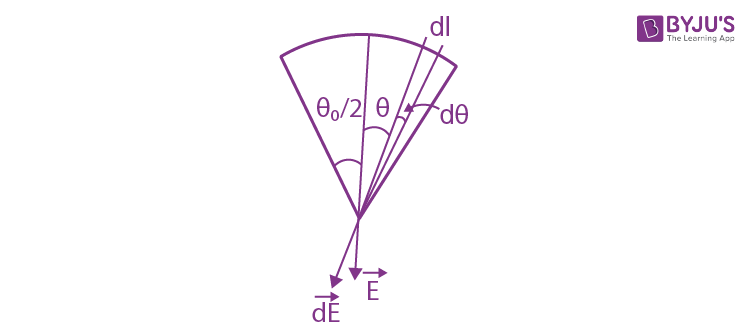From the symmetry the sought field strength will be directed along the bisector of the angle

$$\Theta _{o}$$
and is given by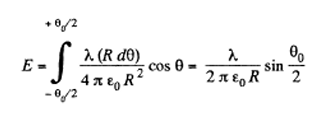In our problem

$$\Theta _{o}=\frac{\Pi }{2}$$
, thus the field strength due to the turned point
$$E_{0}=\frac{\sqrt{2}\lambda }{4\Pi \varepsilon _{0}R}$$
which is also the sought result.

(b)Using the solution of 3.14 (a), net field strength at O due to straight parts equals

$$\sqrt{2}(\frac{\sqrt{2}\lambda }{4\pi \varepsilon _{o}R})= (\frac{\lambda }{2\pi \varepsilon _{o}R})$$

and is directed vertically down. Now using the solution of (a), field strength due to the given curved part (semi-circle) at the point O becomes

$$\frac{\lambda }{2\pi \varepsilon _{0}R}$$

and is directed vertically upward. Hence the sought net field strength becomes zero.

16. A sphere of radius r carries a surface charge of density σ = ar, where a is a constant vector, and r is the radius vector of a point of the sphere relative to its centre. Find the electric field strength vector at the centre of the sphere.

Solution:

1. Given charge distribution on the surface

$$\sigma =\vec{a}.\vec{r}$$
is shown in the figure. Symmetry of this distribution implies that the sought
$$\vec{e}$$
at the center O of the sphere is opposite to
$$\vec{a}$$
.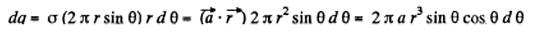Again to from symmetry, field strength due to any ring element

$$\vec{dE}$$

Is also opposite to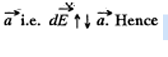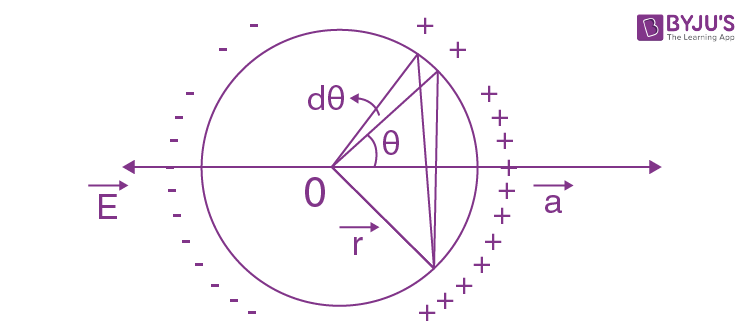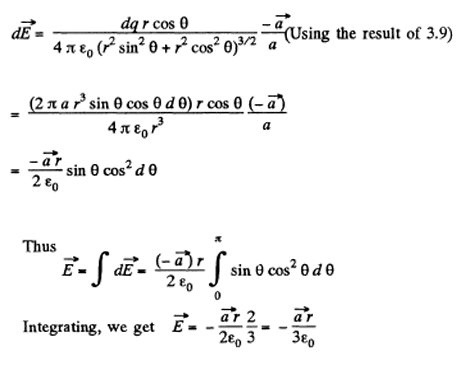17. Suppose the surface charge density over a sphere of radius R depends on a polar angle θ as σ = σ0 cos θ, where σois a positive constant. Show that such a charge distribution can be represented as a result of a small relative shift of two uniformly charged balls of radius R whose charges are equal in magnitude and opposite in sign. Resorting to this representation, find the electric field strength vector inside the given sphere.

Solution:

1. We start from two charged spherical balls each of radius R with equal and opposite charge densities + p and -p at respectively. The centre of the balls are at

$$\frac{\vec{a}}{2} and \frac{\vec{-a}}{2}$$
respectively so the equation of their surfaces are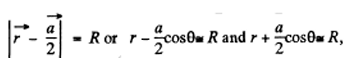Considering a to be small. The distance between the two surfaces in the radial direction at angle

$$\Theta$$
is
$$\left | a\cos \Theta \right |$$
and does not depend on the azimuthal angle. It is sen from the diagram that the surface of the surface has in an effect a surface density
$$\sigma =\sigma _{0}\cos \Theta$$
when
$$\sigma _{0}=\rho a$$

Inside any uniformly charged spherical ball, the field is radial and has the magnitude given by Gauss’s theorem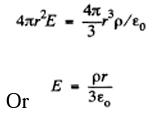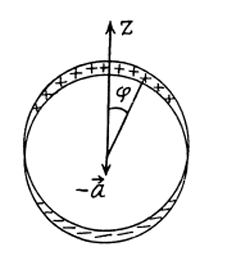In vector notation, using the fact the V must be measured from the centre of the ball, we get, for the present case

$$\vec{E}=\frac{p}{3\pi \varepsilon _{0}}(\vec{r}-\frac{\vec{a}}{2})-\frac{p}{3\pi \varepsilon _{0}}(\vec{r}+\frac{\vec{a}}{2})$$

=

$$-\frac{\rho \vec{a}}{3\varepsilon _{0}}=\frac{\sigma _{0}}{3\varepsilon _{0}}\vec{k}$$

When

$$\vec{k}$$
is a unit vector along the polar axis from which
$$\Theta$$
is measured.

18. Find the electric field strength vector at the centre of a ball of radius R with volume charge density p = ar, where a is a constant vector, and r is a radius vector drawn from the ball's centre.

Solution:

1. Let us consider an elemental spherical shell of thickness dr. Thus surface charge density of the shell

$$\sigma=\rho dr=(\vec{a}.\vec{r})dr$$

Thus using the solution of Q.16, field strength due to this spherical shell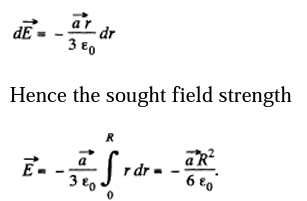19. A very long uniformly charged thread oriented along the axis of a circle of radius R rests on its centre with one of the ends. The charge of the thread per unit length is equal to λ. Find the flux of the vector E across the circle area.

Solution:

1. From the solution of Question.14 field strength at a perpendicular distance r < R from its left end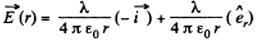$$\hat{e}_{r}$$
is a unit vector along radial direction.

Let us consider an elemental surface,

$$ds=dy dz = dz (r d\Theta ) a$$

figure. Thus flux of

$$\vec{E}(r)$$
over the element
$$d\vec{S}$$
is given by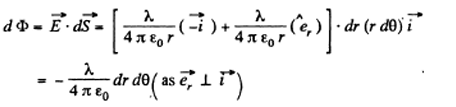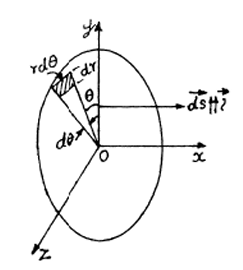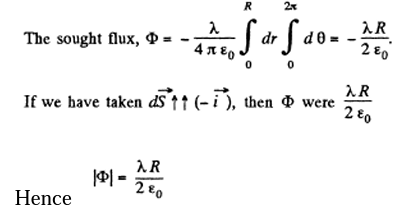20. Two-point charges q and — q are separated by the distance 21 (Figure). Find the flux of the electric field strength vector across a circle of radius R.

Solution:

1.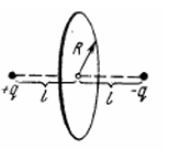Let us consider an elemental surface area as shown in the figure. Then flux of the vector

$$\vec{E}$$
through the elemental area,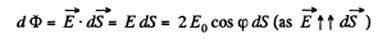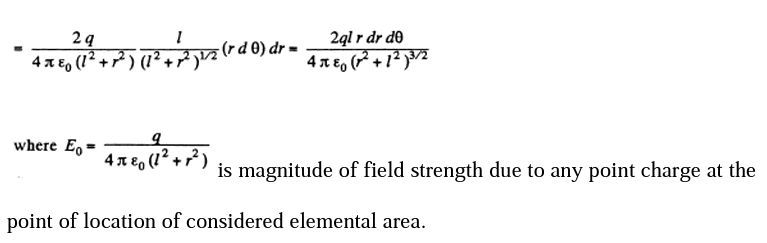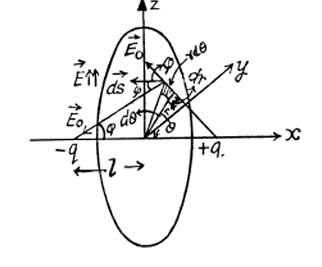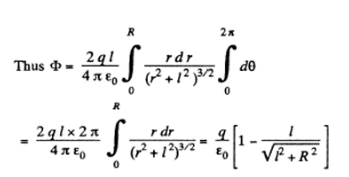We can also solve by considering a ring element or by using solid angle.